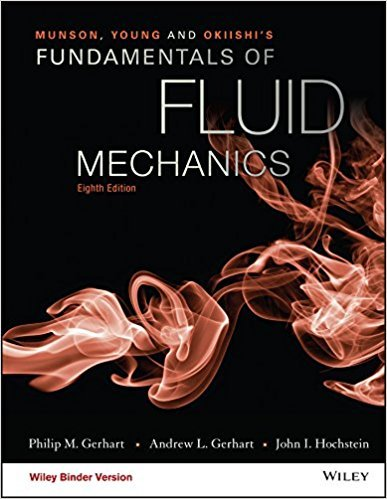×
Get Full Access to Fundamentals Of Fluid Mechanics - 8 Edition - Chapter 7.3 - Problem 7.24
Get Full Access to Fundamentals Of Fluid Mechanics - 8 Edition - Chapter 7.3 - Problem 7.24

×

# A vapor bubble rises in a liquid. The relevant dimensionalparameters are the liquidISBN: 9781119080701 456

## Solution for problem 7.24 Chapter 7.3

Fundamentals of Fluid Mechanics | 8th Edition

• Textbook Solutions
• 2901 Step-by-step solutions solved by professors and subject experts
• Get 24/7 help from StudySoup virtual teaching assistantsFundamentals of Fluid Mechanics | 8th Edition

4 5 1 337 Reviews
17
1
Problem 7.24

A vapor bubble rises in a liquid. The relevant dimensionalparameters are the liquid specific weight, , the vapor specificweight, , bubble velocity, V, bubble diameter, d, surface tension,, and liquid viscosity, . Find appropriate dimensionlessparameters.

Step-by-Step Solution:
Step 1 of 3

How Cells Release Chemical Energy (Respiration) All organisms have to acquire energy: photoautrophs get E (ATP) from the sun heterotrophs get E (ATP) from eating plants and one another ATP - E of the cell - produced during cellular respiration using the mitochondria - the circulatory system diffuses O a2d C H O6to12he6mitochondria for processing  ATP is given off along with CO and H 2 2 - cellular respiration consists of many steps mediated by specific enzymes - high E e’ are removed from glucose breakdown and passed down an E.T.C. to a lower E level - as e’ move from one carrier to the next, E is released and recaptured for ATP production - chemical E (glucose) is transferred into usable E, but some E is lost as heat st nd (1 and 2 Laws of Thermodynamics) - as you exercise, E is produced resulting in a rise in body temperature – sweat cools the body (evaporation, vaporatization) Cellular Respiration C H O + 6 O 6 CO + 6 H O 6 12 6 2 2 2 = a cellular process that requires O a2d gives off CO and 2 O plus2E - opposite of photosynthesis, but don’t forget that plants also use cellular respiration to breakdown sugar to release E glucose = high E carbon dioxide = low E water = low E - when glucose is broken down, E is released (exergonic)

Step 2 of 3

Step 3 of 3

##### ISBN: 9781119080701

The full step-by-step solution to problem: 7.24 from chapter: 7.3 was answered by , our top Science solution expert on 03/16/18, 03:21PM. Fundamentals of Fluid Mechanics was written by and is associated to the ISBN: 9781119080701. This textbook survival guide was created for the textbook: Fundamentals of Fluid Mechanics, edition: 8. This full solution covers the following key subjects: . This expansive textbook survival guide covers 112 chapters, and 1357 solutions. Since the solution to 7.24 from 7.3 chapter was answered, more than 213 students have viewed the full step-by-step answer. The answer to “A vapor bubble rises in a liquid. The relevant dimensionalparameters are the liquid specific weight, , the vapor specificweight, , bubble velocity, V, bubble diameter, d, surface tension,, and liquid viscosity, . Find appropriate dimensionlessparameters.” is broken down into a number of easy to follow steps, and 35 words.

## Discover and learn what students are asking

Calculus: Early Transcendental Functions : Iterated Integrals and Area in the Plane
?In Exercises 1 - 10, evaluate the integral. $$\int_{x}^{x^{2}} \frac{y}{x} d y$$

Chemistry: The Central Science : Introduction:Matter, Energy, and Measurement
?Which of the following figures represents (a) a pure element, (b) a mixture of two elements, (c) a pure compound,

Statistics: Informed Decisions Using Data : Inference about the Difference between Two Medians: Dependent Samples
?In Problems 3–10, use the Wilcoxon matched-pairs signedranks test to test the given hypotheses at the a = 0.05 level of significance. The dependent sa

Unlock Textbook Solution